# Fundamental Statistics for the Behavioral Sciences 9th edition David C. Howell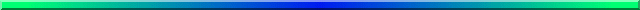Review of Arithmetic:

"I Don’t Do Fractions"

The subtitle tells it all. This was actually the response I received from a student in one of my classes—not a statistics course, by the way. As part of an in-class exercise, they had to convert simple fractions to decimals to calculate their average rating of the quality of chocolate chip cookies. But this student couldn’t handle the arithmetic, and his friends had to help him out.

This episode made me realize that there are some things people like me take for granted, without remembering that there is a whole world out there filled with people who don’t use arithmetic on a daily basis, and have forgotten lots of stuff. I’m not talking about calculus or differential geometry, I’m talking about whether 1/2 + 1/3 = 1/5 or 1/6 or 5/6. (It equals 5/6.) So I have tried to put together a simple review of arithmetic, with a teenie-weenie bit of algebra thrown in for good measure. You may think that some of this material is too simple—I hope you do. But there is probably something here for everyone, since all of us forget even the simplest things when we don’t use them. So I’ll start out with what I hope everyone knows without being told, and progress toward some of the more troublesome topics. In each case I give the answer to one worked example, and then provide a few more examples.

I am not a teacher of arithmetic, and some of the rules that they use everyday, and can pass on to students, are not in my head—they once were, but I forget just like you do. That means that some of my explanations may be a bit clumsy, and may be of the form "well, it works for me." I don’t think there is anything wrong with that. We all develop our own way of looking at things, and perhaps my way will make more sense to you than the rule you learned in school; perhaps it won’t.

Everyone knows how to add, at least if all of the numbers are positive, but I have to start somewhere. Most of the time we deal with positive numbers, because we want to talk about things like the cost of a hamburger and a coke. When we’re dealing with positive numbers we could affix a + sign, but we usually don’t.

(+5) + (+6) = 5 + 6 = 11

(I don’t think I need to supply test items for that!)

But remember, it doesn’t make any difference what order the items are in. If your hamburger costs \$1.50, and your coffee costs \$.80, you don’t really care about the order in which the clerk adds them up.

1.50 + .80 = .80 + 1.50 = \$2.30

But what about negative numbers? Well, subtraction is really just adding negative numbers, so I might as well cover them here? Suppose that you had a discount coupon worth \$.25 that you used for your hamburger and coffee. Then you can just add that in too, affixing a minus sign.

1.50 + .80 + (-.25) = 1.50 + .80 – .25 = \$2.05

So, to add negative numbers, you really just subtract the negative numbers from the positive ones.

To make this a bit more complicated, assume that your girl friend also had a coupon, though hers was worth \$.40. You could either just add (and subtract) things as you go along, as in

1.50 + .80 + (-.25) + (-.40) = 1.50 + .80 - .25 - .40 = \$1.65

or you can first collect terms having the same sign.

1.50 + .80 + (-.25) + (-.40) = 1.50 + .80 - .25 -.40 =

(1.50 + .80) – (.25 + .40) = 2.30 - .65 = \$1.65

Notice that I added together the positive items (the food), I added together the negative items (the coupons), and then I subtracted the negative sum from the positive sum.

Examples

2 – 1 + 5 – 3 – 4 + 8 = ?

5 + 4 – 3 – 2 + 4 – 2 = ?

12 + 14 – 6 + 3 – 10 = ?

What about 5 – 8 + 6? You probably don’t have any trouble with that, even though if you do it sequentially you have to go from 5 to –3 to +3. If those were much larger numbers, you could still do it the same way, but I prefer to collect terms with the same sign, add those two groups up, and then subtract the sum of the negative numbers from the sum of the positive ones. For example, add the 5 and 6 to get 11, and then subtract the 8 to get 3; i.e. 5 + 6 = 11, 11 – 8 = 3.

But what if the negative numbers are larger than the positive ones? For example, what about 23 – 36.

That’s easy, too. Just do the subtraction in reverse, and then reverse the sign. In other words, instead of subtracting 36 from 23, subtract 23 from 36, but make the answer negative.

23 – 36 = –(36 – 23) = –13.

Examples

8 – 9 = ?

17 – 22 = ?

54 – 87 =?

Parentheses

Parentheses are our friends. They make things so much clearer, and it is very hard to go wrong with them if you follow a simple rule. Always work from the inside out!

One of the big advantages of parentheses is that they tell us what order to do things in. For example, if you wanted to add 8 and 9, and then divide the answer by 3, you cannot write 8 + 9/3, because that would tell you to add 8 to the result of 9/3, which would be 11. Instead we put the parentheses around 8 + 9, and then show the division by 3, as in
(8 + 9)/3 = 17/3 = 5.667.

As an aside, you do the same thing when you are playing on the Internet. If you want to look up information on George Washington or Abe Lincoln, you might type in "George and Washington or Abe and Lincoln." However, your software, taking you very literally, would look for documents that contain all the names George and Washington and Lincoln, or the name Abe. That’s not what you wanted. You wanted (George and Washington) or (Abe and Lincoln). Notice how the parentheses keep things together that belong together? They do the same thing in arithmetic.

A big thing about parentheses is the way that they deal with signs—particularly minus signs. In an earlier example I went from 23 – 36 to – (36 – 23). How did I do that? Well, I did it by knowing that when I have a negative outside a set of parentheses, and I remove the parentheses, I just reverse all the signs. For example,

–(23 –14 + 16 – 5 + 8 + 7) = –23 + 14 – 16 + 5 – 8 – 7

This rule only indirectly answers the question I asked. I asked how I could go from 23 – 36 to – (36 – 23), but I answered by going from –(36 – 23) to –36 + 23 to 23 - 36. Well, that’s because I couldn’t think of a rule to say it the other way around. See if you can come up with one. The way I did it works, it’s just a bit clumsy.

About half way through the book you are going to come across a formula like the following. z = (x̄ – μ)/(σ /√N) This formula doesn’t have any numbers in it, only symbols, so it is really algebra instead of arithmetic. But you interpret it the same way you interpreted the examples above. First you subtract μ from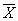. Then you divide σ by the square root of N. Then you take the first answer and divide it by the second. All that I am doing is worrying about what comes inside parentheses before I worry about what is outside.

It gets even more fun.

The above example had two sets of parentheses. What if you have one set nested within another? For example,

(8 + (5 + 1)/2)/7

You follow the same rule. Start inside the inner parentheses and add 5 + 1 = 6. Then do the stuff within the next set of parentheses, which tells you to divide 6 by 2  and add 8. This gives you 8 + 3 = 11. Finally, divide that by 7, getting 1.57.

With nested parentheses we always work from the inside out. That is true if we have truly nested parentheses, as in 4 × ((8 + 4)/3 – (6 + 3)/3) = 4 × (4 – 3)= 4(1) = 4, or if you use a mixture of parentheses and brackets, as in 4 × [(8 + 4)/3 – (6 + 3)/3)]. Brackets, parentheses, and braces "{ }" mean the same thing. We just sometimes mix them up so that our eyes don’t get crossed trying to figure out which parenthesis goes with (closes) which other parenthesis.

Try the following example. Note: treat a square root sign like a set of parentheses—i.e. do whatever is under the square root sign before you take the square root.

Example

How would you carry out the following steps, assuming that you knew what the symbols meant?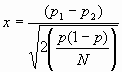Multiplication and Division

I show multiplication in several different ways in the text. For example, I could write 2 × 3, or (2)(3), or 2(3), or 2*3. The latter is something that copy editors wrap my knuckles over, but some probably snuck through. They all mean the same thing.

Similarly in division, we can write 1/2, or ½, or 1÷2. Again, they all mean the same thing, although the last one isn’t used much anymore.

Multiplication by 0 leaves 0 as an answer.

5 × 0 = 0

If you didn’t have any money yesterday, and you have 5 times that much today, you’re still broke.

Multiplication by 1 doesn’t change anything.

1 × 5 = 5

You haven’t multiplied it by anything, so it hasn’t gotten any bigger.

Division by 1 doesn't change anything.

5/1 = 5.

A \$5 bill cut into one piece is still a \$5 bill.

Division by zero is infinity, or undefined, depending on what you want to call it.

5/0 = ∞

If you had \$5 yesterday and you chopped it up into 0 pieces, what have you got? Beats me! We try very hard never to get an equation that works out to having 0 in the denominator, because that brings us to a big halt.

Division of 0 by something is 0

0/5 = 0

If you didn’t have any money yesterday, and you chopped that empty wallet into 5 pieces, it still doesn’t have any money in it.(But that leaves the interesting question of what happens if you divide 0 by 0. Hmmm! There must be more important things to worry about.)

Multiplying positive and negative numbers.

This one is easy. When you multiply some positive numbers and some negative numbers together, the answer is positive if there are an even number of negative signs, and the answer is negative if there are an odd number.

-2 × 3 = -6

-2 × (-3) = 6

-2 × 5 × (-8) × (-3) × 3 = -720

Examples

3 × (-5) = ?

-5 × (-8) × (-2 ) × 4 = ?

8 × (-2) × (-3) = ?

9 × (0/5) = ?

7/1 + 12 = ?

9 × (5/0) = ?

(-9 × 12)/3 = ?

Dividing positive and negative numbers.

The logic here is the same as it was above. If there on an odd number of negative signs, the answer is negative; otherwise it is positive.

4/(-3) = -1.333

-4/3 = -1.333

-4/(-3) = 1.333

Examples

8/(-2) = ?

12/6 = ?

18/(-4) = ?

-15/3 = ?

-25/(-5) = ?

Fractions

Here is where students get confused. They know that there are rules, but they don’t know what those rules are, so they give up. I’ll supply a few rules in a minute, but it is very much more important for you to realize that you can figure out what those rules are in about 15 seconds.

Before I go any further, however, I have to remind you about numerators and denominators. The numerator is the thing on the top, and the denominator is the thing on the bottom. So in 5/7, the 5 is the numerator and the 7 is the denominator. You can remember this because the "de" prefix is found in words like "denounce," "denigrate," and "deficit," all of which have a connotation of "put down," which reminds you of putting something on the bottom.

If I gave you 1/5 + 2/5 and asked you what it was, you might have a problem. (I hope you wouldn’t, but bear with me.) But I’m sure that you know that 1/3 plus 1/3 is 2/3. From that you ought to be able to figure out whether you are supposed to add the numerators, or add the denominators, or add both. The only way that you could get from 1/3 + 1/3 to 2/3 is to add the numerators but not the denominators. (If you added both numerator and denominator, you would have 2/6, which is just 1/3, so that can’t be right. If you add just the denominators you would get 1/6, and that is less than each of the pieces, so it can’t be right either. That only leaves you with the possibility of adding the numerators, which does give the right answer.)

The rule is that if two or more fractions have the same denominator, you just add up the numerators and put that sum over the common denominator. So

1/5 + 3/5 = 4/5.

1/8 + 2/8 + 3/8 = 6/8.

5/8 + 7/8 = 12/8 = 1 and 4/8 = 1.5

Examples

1/3 + 2/3 = ?

2/7 + 3/7 = ?

8/7 + 3/7 = ?

8/7 – 5/7 = ?

Notice that I snuck in a subtraction for the last one. You should be able to figure out that if in addition you add the numerators, in subtraction you must subtract them.

4/5 – 3/5 = 1/5

6/9 – 2/9 = 4/9

4/9 – 7/9 = -3/9

Examples

3/5 – 1/5 = ?

4/7 – 1/7 = ?

12/7 – 6/7 = ?

10/6 – 15/6 = ?

Things get messy when you have different denominators. I just said that if you have the same denominator, you can add (or subtract) the numerators. But what if the denominators are different?

Well, we won’t let you have different denominators. If they are different, you’ll just have to make them the same before you go on. So, if you want to add 1/2 and 1/3, you’ll have to find a way to express both of those with the same denominator. Certainly you know that 1/2 = 3/6, and that 1/3 = 2/6, so just convert the problem from 1/2 + 1/3 to 3/6 + 2/6 = 5/6

(Once you have the common denominator, then you can go ahead and add the numerators.)

Examples

1/2 + 3/4 = ?

4/5 + 3/2 = ?

3/4 – 2/8 = ?

The system above works just fine if you have things like 3/4 and 2/3, but what about 7/22 + 3/17? Well, there is a rule for doing that, but I’m not going to try to get you to use it, because I can’t think when you would want to. The way that most people do this is to convert each to a decimal, and then add the decimal equivalents.

7/22 = .3182

3/17 = .1765

.4947

But how did you do that?

Here is where the student mentioned at the beginning of this section fell apart. He apparently didn’t remember how to get from 7/22 to .318. Well, you just have to remember that 7/22 really means 7 divided by 22, and if you can’t do that division on paper, you can certainly do it on a calculator. I’m not going to try to teach you to do long division.

Examples

4/9 + 5/7 = ?

15/33 + 5/7 = ?

3/6 + 4/9 = ?

Multiplying fractions

When you multiply fractions, you just multiple the numerators together and the denominators together.

1/2 × 1/2 = 1/4 , which is what you would expect.

2/3 × 4/5 = 8/15

7/3 × 6/2 = 42/6 = 7

Examples

2/3 × 1/2 = ?

4/5 × 3/4 = ?

7/8 × 6/13 = ?

Notice that in each of the answers I have "reduced the fraction" when I could. Since both the numerator and the denominator in 2/8 can be divided by 2, I can do that to get 2/8 = 1/4. It doesn’t hurt if you don’t, but it is much neater if you do. (Who wants to look at 21/49 when they can divide both by 7 and get 3/7?)

Multiplying signed fractions

What if we have fractions with minus signs attached? For example

-3/4 × 2/5 = -6/20 = -3/10

You just do exactly the same thing as you do when you multiply whole numbers. If the number of minus signs are even, the answer is positive. If the number of minus signs are odd, the answer is negative.

Examples

-3/5 × 2/3 = ?

-3/7 × -4/9 = ?

-1/3 × 3/4 × -3/8 = ?

-1/2 × 2/3 × 4/5 = ?

Multiplying a fraction by a whole number

What about 2 × 4/5? Well, that is really just 2/1 × 4/5, so you multiply the numerators and multiply the denominators, which gives (2 × 4)/(1 × 5) = 8/5, which is the same as just multiplying the numerator by the whole number.

What if the multiplier is a decimal?

Nothing changes. Just multiply the numerator by the decimal.

0.4 × 3/7 = (0.4 × 3)/7 = (1.2)/7

Dividing fractions

Here things could get a bit messier. What about (2/3) / (3/4)?

There are a couple of ways you could do this. You could start by converting each fraction to a decimal, and then dividing the two decimals.

>(2/3) / (3/4) = 0.6667 / 0.75 = 0.8889
Emily Todicescu pointed out that I had a completely wrong answer here. I told you that it is easy to mess up with simple arithmetic.

That will always work, but it is bit on the overkill side if you have nice tidy fractions. What I do is to mentally invert the denominator, and then multiply. (By "invert the denominator" I mean "flip it upside down."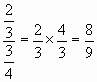That will work with even messy fractions, but for messy fractions it is easier to get the decimal equivalents first.

Examples

(1/3) / (2/3) = ?

(5/6) / (8/7) = ?

(12/7) / (7/39) = ?

Algebraic fractions

The same rule that applies to numerical fractions also applies to algebraic ones. For a one-sample t test we are going to have something like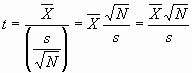Notice that I have just inverted the denominator and multiplied it by the numerator. There are very few times when you would have to do something like that with this text, but I thought I would sneak in a little aside. Notice that you don’t even have to know what any of those symbols in the equation represents to be able to do the manipulation.

Decimals and Rounding

Many of the numbers we will deal with are decimals, and we don’t usually want to carry around all of those decimal places. This leaves us with two questions. How many decimals should we carry, and how should we round up or down to them.

Decimals

There are all sorts of rules about how many decimal places to carry, and whatever I say will upset someone. So I’ll just go ahead and give an answer without trying to defend it. (I’d probably have a hard time defending it to a mathematical purist.)

If the numbers you are working with are whole numbers, carry 2 or three decimal places. Thus represent 234.6778767 as 234.68 or maybe 234.678

If you have very small numbers, keep 1 to 3 non-zero decimals. Thus represent .00044178 as .0004 or .00044.

There are lots of places in the book where I violate these rules, sometimes for good reasons, and sometimes because I am careless. But the rules are at least rough guidelines to go by.

Rounding

There is not only continual dispute over how many decimal places to carry, but also over how to cut down to that many decimal places. I am going to state a very simple set of rules.

If you are more than half way toward the next number, round up.

If you are less than half way toward the next number, round down.

If you are teetering one the exact center, make the result even.

Suppose that we have the number 2567.45654 and we want to round it to a whole number. You are somewhere between 2567 and 2568, and half way would be 2567.500000000. So you are less than half way to 2568, so round down to 2567..

But suppose you had 2567.656782. Here you are more than half way toward 2568, so round up. I would do this even if you had 2567.50002—It is still more than half way to 2568.

Now suppose that you had 2567.500000. Here you are exactly half way in the middle. You aren’t any closer to 2567 than to 2568. My rule is to make the result even, so I would round up to 2568. But, if I had been at 2566.50000, I would have rounded down to 2566 to make the result even.

The only time when you try this odd/even trick is when you are exactly on the border. If you are closer to one end or the other, go to that end. The odd/even bit means that you will round up about half the time and round down about half the time. What could be fairer?

The same rules apply no matter how many decimal places you chose to have. So, if you want to carry 3 decimals, then

2658.5678 ⇒ 2658.568

2658.5672 ⇒ 2658.567

Squares

In statistics we often square values, or take their square root, which I’ll discuss next. Squaring a value simply means multiplying the value by itself, and is represented with a superscript 2. So, X squared = X × X = X2. Then, 42 = 4 × 4 = 16.

If you have a slightly more complex expression, such as 5X2, you square X before you multiply by 5.

Finally, raising something to some other power is the same basic idea. The cube of a number is that number raised to the third power. So X3 = X × X × X.

Examples

62 = ?

8(32) = ?

0.52 = ?

Squares of expressions

Remember the rule about parentheses that I gave you earlier. If you have an expression like (5 + 12)2, you do the stuff within the parentheses before you do the stuff outside the parentheses. So you first add 5 and 12 to get 17, and then you square 17, which is 172 = 289. It is definitely not 52 + 122 = 25 + 144 = 169. Always do what is within parentheses before you do anything else.

Square roots

The square root of the number is just that value which, when squared, equals the number. For example, we know that 52 = 25, so √25 = 5; The thing over the 25 is called a radical, and is a square root symbol. (Unless you have an equation editor, it is hard to make a proper radical on a word processor. The closest we can come is the √ symbol. When you see √25.7, it means the same as √25.7 . You just have to pretend that the bar extends over the digits.)

Once upon a time, when I was a little boy in about 7th grade, I was taught how to take the square root of any number with pencil and paper. You set the problem up sort of like a long division problem, did a bit of magic, and there was your answer. I was pretty good at everything but the magic bit, but that I never could work out. Well, you’re in luck. You have a calculator, and it has a square root key, and that’s how we find square roots these days.

Technically, 25 actually has 2 square roots. 52 = 25, and (-5)2 = 25, but in statistics we always use the positive square root of a number.

Just as we do things within parentheses, and then square the result, when getting square roots we do what is under the radical before we take the square root. So, √18+7 = √25 = 5. We do the addition first.

Percentages and Proportions

Percentages and proportions are very similar, and sometimes get people confused. In fact, a percentage is really just a proportion times 100. If your class has 32 students and 29 did well on the last exam, then the proportion of those doing well is 29/32 = .91. That means that 91% of the students did well.

To make things even messier, we sometimes express a probability as a percentage—which is really bad. A probability is a number between 0 and 1. I can say that the probability of rain is .35, but I should never say that it is 35%. Of course, I do say just that on occasion, but that doesn’t make it right.

Don’t let yourself get confused between percentage and percentage points. Suppose that for your class of 32 students, 25 did well on the first exam and 29 did well on the second. Converting to percentages we have 78% and 91% doing well on the two exams. The percentage of students doing well increase 13 percentage points, from 79% to 91%. But that is a (29 – 25)/25 = 4/25 = 16 percent increase in the number of students doing well.

Inequalities

You will frequently come across inequalities in this book. They are things like less than (<), less than or equal to (<), greater than (>), and greater than or equal to (>). The most frequent use of an inequality that you will see is the expression p < .05, which translates to "the probability is less than .05." Sometimes you will see p > .05, which obviously means that the probability is now greater than .05.

Another of the expressions you will see is something of the form 25 < X < 75. This really means that some quantity we call X is between 25 and 75. (It is read "25 is less than or equal to X, which is less than or equal to 75." When you see multiple sets of < like this, just read it as "between."

Absolute values

I will occasionally refer to absolute values, symbolized by something like |4|. This simply means to ignore whatever sign there is for 4, which is basically the same as saying "treat 4 as positive."

|4| = |-4| = 4

|6-9| = 3

The most common way in which you will see absolute values is in the statement.
|X|. This says to subtractfrom X, throw away the minus sign if there is one, and treat the answer as positive.

You will also come across a statement like p(|z| ) > 1.96 = .05. This translates to "the probability that the absolute value of z will be greater than 1.96 is .05." That, itself, translates to "the probability is .05 that z will be more positive than 1.96 or more negative than –1.96."

Equations

We have already been working with equations, but I should put a few things in a section devoted to equations. The material here is material that you can expect to see in the text. I am leaving out topics that I don’t think you are likely to come across.

An equation is an expression with an equal sign separating two quantities. Thus

Y = bX + a

is an equation that says that the thing on the left (Y ) is equal to the expression on the right. You probably already knew that. But what about a few things that you might have forgotten?

If you do the same thing to both sides of the equation, you haven’t really changed anything.

For example, you can add 12.6 to both sides of the equation, and the equality is still true.

Y + 12.6 = bX + a + 12.6

If you can add, you can also subtract, because subtraction is just addition of a negative number.

You can multiply (or divide) both sides of the equation by the same thing without changing the equality.

3 × Y = 3(bX + a)

That basic rule tells us a lot about how to solve equations.

For example, suppose that we have

3Y = 7X + 8.

If we divide both sides by 3 we have

3Y/3 = (7X +8)/3

Therefore

Y = (7X +8)/3

In my own head I think of it slightly differently. If there is a multiplier on one side of the equation, I move it to the other side as a divisor, Similarly, if there is a divisor on one side, I think of moving it to the other side as a multiplier. But I am doing exactly what this rule says, I’m just not thinking about it in that way.

A similar rule applies to addition and subtraction. Take the same example:

3Y = 7X + 8.

3Y – 8 = 7X

I just moved the 8 to the other side and made it negative. Or, if you prefer the more proper interpretation, I just subtracted 8 from both sides of the equation.

Now let’s put the two together.

If I want to solve for X, I must get rid of that 8. I did that in the last step. Then I get rid of the 7 by dividing.

3Y = 7X + 8.

3Y – 8 = 7X

(3Y –8)/7 = X

One more complication.

Suppose that we have one side of an equation squared. For example, you will see expressions for the variance and the standard deviation, one of which is just the square of the other.

The variance is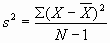The standard deviation is the square root of the variance, so we just take the square root of both sides. The square root of s2 is s, and will just put a radical around the right side to show that as a square root.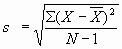(Radicals are a nuisance to deal with, so you will often see statisticians square both sides of the equation before carrying out other operations.

Conclusion

I have covered the very basic material here, but what I covered should prepare you for just about anything you will see in the book. By far the most important thing to take away from this section is the idea that if you don’t know how to do something, make up an example with very simple numbers, and play with it. When you figure out how to make the simple numbers come out right, you will have also figured out how to make the more complicated stuff come out right.

There is another way that is really important. ASK GOOGLE!! Just type in "How do I multiply fractions?" and I guarantee that you will get at least once answer that is sensible and easy to understand. Don't ever forget that Google is your friend!!

If there is something that I have left out, please let me know. Web sites can be updated daily, and it is easy to do.

Finally, if you want more review, try the web. There is a very good site at http://www.icmicroanalysis.com/mathtutorials.htm . Just scroll to the middle of the page and there are a bunch of good links--though the level of difficult varies.dch:

David C. Howell
University of Vermont
David.Howell@uvm.edu